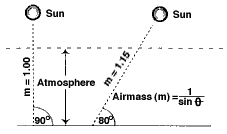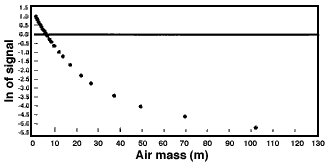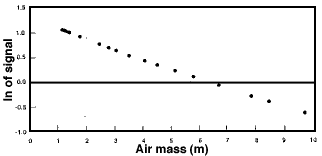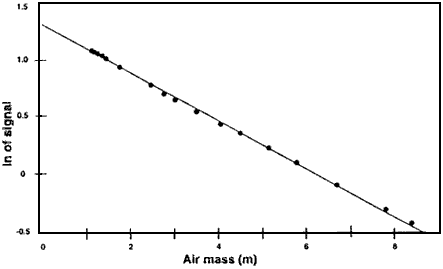##Hands On Physics

### Haze Concepts CALIBRATION DATA ANALYSIS

There are several ways to analyze your data to find the extraterrestrial or ET constant, all of which should yield the same result.
After you find your instrument's ET constant, you will insert it in a formula that will give you a standardized measurement of haze known as aerosol optical thickness (AOT).
When you made your Langley measurements for a range of Sun angles, each time the angle changed the thickness of the atmosphere (the air mass) between you and the Sun changedFigure P2
Air Mass

When the Sun is straight overhead, its light passes through one thickness of atmosphere or one air mass. The air mass (m) increases as the Sun moves closer to the horizon. The m for each of your measurements is 1 divided by the sine of the Sun's angle

If you make a graph of the natural logarithm (ln) of the signal you measured versus m when you made the measurement, the data points should fall along a straight line out to an m of 10 or so, assuming the sky conditions were stable during your measurement period. If you extend the straight line, the ET constant will be where the line crosses the air mass axis at m = 0. When m = 0, there is no air mass, which means this is the signal the TERC VHS-1 will give if you could take it to the top of the atmosphere.

Several methods are available for extracting the ET constant from your Langley test data. They should all give similar or nearly identical results. All three methods perform what is called a linear regression on your data. Briefly, a linear regression fits the equation for a straight line to your data. In this case, ln of signal = a + (b x air mass).
You can use this equation to find the signal for m = 0.

### Graph Paper Method

• Make a table of the TERC VHS-1 measurements for a range of air masses up to about 10. The first column should be the air mass (m) for each measurement and the second column the Sun signal - the dark signal.
• Add a third column to your table for the natural logarithm (ln) of the signal. (Use a scientific calculator with a ln key.)
• Print this title on the abscissa (x or horizontal axis) of the graph: Air mass (m).
• Print this title on the ordinate (y or vertical axis) of the graph: ln of signal.
• Label the major divisions of the abscissa 0 to 10, as shown in Fig. 7. <P3>
• Plot the pairs of data in the first and third columns on your table on the graph.
• Use a straight edge to draw a line through the center of the points. Extend the line to the ordinate as shown in Fig. 7. <P3>
• The ET constant is where the line crosses the ordinate. Find the value at this point and write it on your graph as you will need it for the haze formula. The ET value derived from the hand drawn graph in Fig. 7 <P3> is based on the first Langley test made with a prototype TERC VHS-1. The ET constant is 1.292, which is very close to the values given by the calculator and a computer program.

### Scientific Calculator Method

• Make a table of the TERC VHS-1 measurements for a range of air masses up to about 10. The first column should be the air mass (m) for each measurement and the second column the Sun signal - the dark signal.
• Add a third column to your table for the natural logarithm (ln) of the signal. (Use a scientific calculator with a ln key.)
• Enter the data pairs into a scientific calculator capable of performing a linear regression.
• Insert the coefficients given by the calculator to calculate the ln of the signal when m = 0. This number is the ET constant, so write it on your table. The ET constant for the prototype TERC VHS-1 is 1.2946.

### Computer Spreadsheet Method

• The TERC VHS-1 spreadsheet program (5) automatically determines the air mass for each data point. You can then use a scientific calculator, the spreadsheet's regression function or curve-fitting software to find the formula for the best fit through the points. The TERC VHS-1 spreadsheet can be downloaded from: ftp://ftp.concord.org/pub/haze/vhs1-download/

• Figure P4 below shows a computer-generated graph of the all the data from the first Langley test of a TERC VHS-1. Notice how the data begin to curve when the air mass exceeds about 10? This established that the upper air mass limit for this instrument is m = 10.

•Figure P4
Langley Plot

• The next figure shows a second computer-generated graph for only the data up to m = 10. Notice how the points appear to form a straight line.

•Figure P5
A Part of the Langley Plot

• The graph below shows that the points in Figure P5 form a very straight line indeed. This graph was made with a powerful curve-fitting program called TableCurve (Jandel Scientific). In only seconds, this program fitted the data to hundreds of mathematical functions. It then drew the graph below in Fig. P6 for the straight line formula (y = a + bx).

•Figure P6
Fitting the Curve

• The program also gave the coefficients for the formula and the value of the Sun signal for m = 0 (the ET constant). The ET constant for the prototype TERC VHS-1 is 1.2946, the same number provided a little more slowly by a standard scientific calculator using the same data.

Previous Page || Up a Level || Index || Next Page